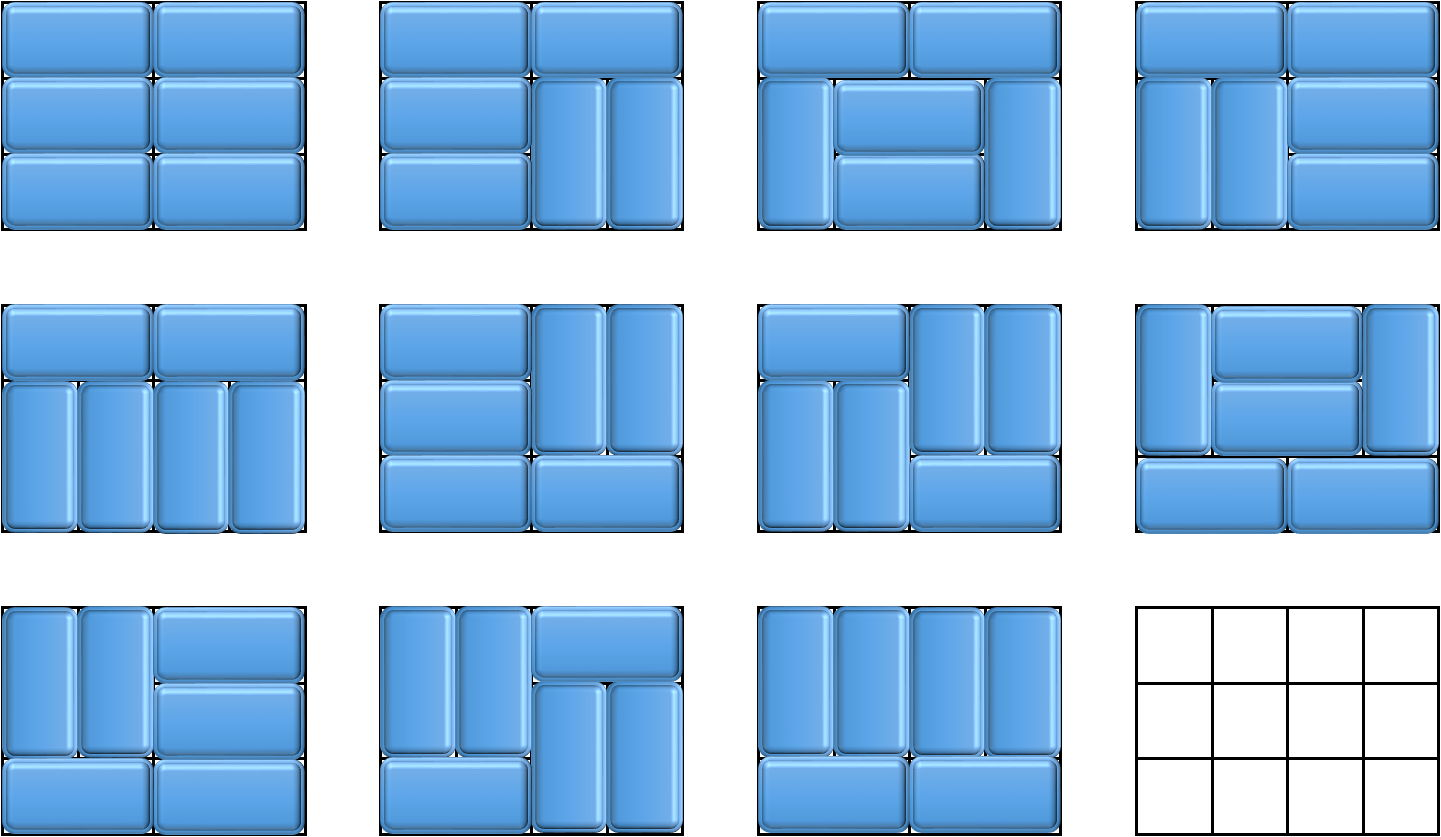시간 제한메모리 제한제출정답맞힌 사람정답 비율
6 초 512 MB3262482.878%

## 문제

Elizur has an empty n × m grid, and he wants to use some 1 × 2 and 2 × 1 dominoes to cover the entire grid. In the grid, each domino ought to cover exactly two adjacent squares and each square ought to be covered by exactly one domino. Two squares are adjacent if and only if they share a common side.

Obviously, he can achieve that if and only if at least one of n and m is even: otherwise, there is always a square that must be left empty. Hence, he wants to know in how many ways he can cover the entire grid. Two ways are considered different if and only if there exist two dominoes, one from the first covering and one from the other, such that one of the squares cover is the same but the other is different.

Can you help him determine the answer? The answer may be exceedingly large, so he only asks you to find it modulo a prime number p

## 입력

The first line contains a single integer T (1 ≤ T ≤ 20 000), indicating the number of questions.

Each of the next T lines contains three integers, n (1 ≤ n ≤ 35), m (1 ≤ m ≤ 1018), and p (2 ≤ p ≤ 230, p is prime), describing one question.

It is guaranteed that no more than 1000 cases satisfy n > 5 or m > 109.

## 출력

For each question, output a single line with a single integer: the answer modulo p.

## 예제 입력 1

6
2 2 23
2 3 233
3 3 2333
3 4 23333
4 4 2332333
5 251346744251346744 998244353


## 예제 출력 1

2
3
0
11
36
295381485


## 힌트

The following image shows all possible ways (11 in total) for the 3 × 4 grid.